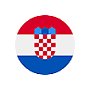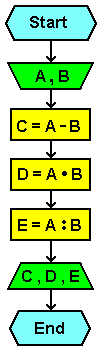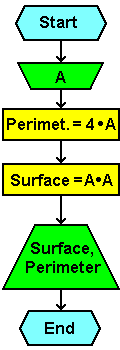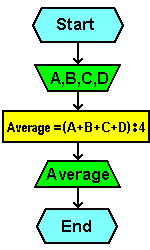# 6.2.4. Algorithm, flowchart and program codeThe following example shows the ten simple tasks that are making the FLOWCHART and code in QBASIC by default algorithm. To create a FLOWCHART used graphic forms are shown in Figure 3.4.6. Logic flow diagram elaborates algorithm that describes the task of program support, and basically they are drawn paths (flow) and the conditions under which the program can be run, a graphical display of cause-effect relationships in a program created by the aforementioned graphical form.

## Example I

Write an algorithm and flowchart for calculating the difference, and the quotient the product of two given numbers.

Algorithm:

```start
-----------------------------------------------------
Input A, B
C = A - B
D = A × B
E = A / B
Print C, D, E
-----------------------------------------------------
end
```
 Flowchart: Program code:``` CLS CLEAR INPUT a, b c = a - b d = a * b e = a / b PRINT c, d, e END ```## Example II

Write an algorithm and flowchart for calculating the perimeter and surface of square, if the default length of the sides of the square.

Algorithm:

```start
-----------------------------------------------------
Input A
PERIMETER = 4 × A
SURFACE = A × A
Print PERIMETER, SURFACE
-----------------------------------------------------
end
```
 Flowchart: Program code:``` CLS CLEAR INPUT a per = 4 * a sur = a * a PRINT per, sur END ```## Example III

Write an algorithm and flowchart for computing the average number of the default 4 numbers.

Algorithm:

```start
-----------------------------------------------------
Input A,B,C,D
AVERAGE = (A + B + C + D) / 4
Print AVERAGE
-----------------------------------------------------
end
```
 Flowchart: Program code:``` CLS CLEAR INPUT a, b, c, d avr = (a + b + c + d) / 4 PRINT avr END ```Citing of this page: Radic, Drago. " IT - Informatics Alphabet " Split-Croatia. {Date of access}. . Copyright © by Drago Radic. All rights reserved. | Disclaimer